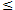# PSAT/SAT & ACT Math Attack – The Greatest IntegerThis week’s PSAT/SAT & ACT Math Attack focuses on the values of integers, with particular attention being given to the values of negative integers. If you’re not careful, it’s very easy for a mental error to cause you to miss an otherwise easy question. This week’s question is a great example of a question that, if you’re not paying attention, is easy to miss.

1. If [x] is the greatest integer less than or equal to x, what is the value of [-1.6] + [3.4] + [2.7]?
1. 3
2. 4
3. 5
4. 6
5. 7

Before attempting to answer the question, you must understand [x] is an operation:

[x] = (Greatest Integerx)

[x] is representative of the respective values of -1.6, 3.4, and 2.7, and for each of those values [x] must be an integer, which is a positive or negative whole number, and that integer must be less than or equal to the values of -1.6, 3.4, and 2.7.

The first thing you can do is disregard the verbiage “equal to.” Though -1.6, 3.4, and 2.7 are all real numbers, none of them is an integer as they all have decimal points. In light of this, we are now looking for the integer for each number that is less than but closest to that number in value.

The respective values of [x] for each of these numbers is

[-1.6] = -2 *Remember: in the case of negative numbers, a smaller number (i.e. a number that is closer to zero) would actually be greater in value, not less than.

[3.4] = 3

[2.7] = 2

After arriving at these numbers it’s a simple matter of plugging them into the equation and solving:

[-1.6] + [3.4] + [2.7] = -2 + 3 + 2

[-1.6] + [3.4] + [2.7] = 3